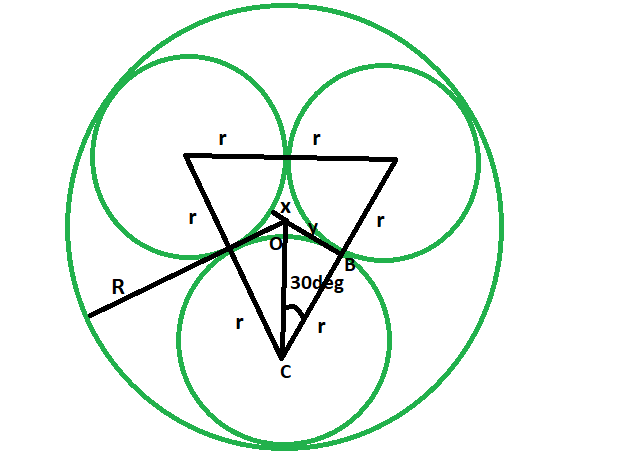# Radii of the three tangent circles of equal radius which are inscribed within a circle of given radius

Given here is a circle of a given radius. Inside it, three tangent circles of equal radius are inscribed. The task is to find the radii of these tangent circles.

Examples:

```Input: R = 4
Output: 1.858

Input: R = 11
Output:: 5.1095

```Approach:

• Let the radii of the tangent circles be r, and the radius of the circumscribing circle
is R.x is the smaller distance from the circumference of the tangent circle and the center of the circumscribing circle.
• From the diagram, it is very clear,
2r + x = R
• now in triangle OBC,
cos 30 = r/(r+x)
rcos30 + xcos30 = r
x = r(1-cos30)/cos30
• also, x = R-2r
• So,
R-2r = r(1-cos30)/cos30
R-2r = 0.133r/0.867
R-2r = 0.153r
R = 2.153r

so, r = 0.4645R## C++

 `// C++ program to find the radii ` `// of the three tangent circles ` `// of equal radius when the radius ` `// of the circumscribed circle is given ` ` `  `#include ` `using` `namespace` `std; ` ` `  `void` `threetangcircle(``int` `R) ` `{ ` `    ``cout << ``"The radii of the tangent circles is "` `         ``<< 0.4645 * R << endl; ` `} ` ` `  `// Driver code ` `int` `main() ` `{ ` `    ``int` `R = 4; ` `    ``threetangcircle(R); ` `    ``return` `0; ` `} `

## Java

 `// Java program to find the radii ` `// of the three tangent circles ` `// of equal radius when the radius ` `// of the circumscribed circle is given ` `import` `java.io.*; ` ` `  `class` `GFG  ` `{ ` ` `  `static` `void` `threetangcircle(``int` `R) ` `{ ` `    ``System.out.print(``"The radii of the tangent circles is "` `        ``+ ``0.4645` `* R); ` `} ` ` `  `// Driver code ` `public` `static` `void` `main (String[] args)  ` `{ ` `    ``int` `R = ``4``; ` `    ``threetangcircle(R); ` `} ` `} ` ` `  `// This code is contributed by anuj_67.. `

## Python3

 `# Python3 program to find the radii ` `# of the three tangent circles ` `# of equal radius when the radius ` `# of the circumscribed circle is given ` ` `  `def` `threetangcircle(R): ` `    ``print``(``"The radii of the tangent"``, ` `          ``"circles is "``, end ``=` `""); ` `    ``print``(``0.4645` `*` `R); ` ` `  `# Driver code ` `R ``=` `4``; ` `threetangcircle(R); ` ` `  `# This code is contributed  ` `# by Princi Singh `

## C#

 `// C# program to find the radii ` `// of the three tangent circles ` `// of equal radius when the radius ` `// of the circumscribed circle is given ` `using` `System; ` ` `  `class` `GFG  ` `{ ` ` `  `static` `void` `threetangcircle(``int` `R) ` `{ ` `    ``Console.WriteLine(``"The radii of the tangent circles is "` `        ``+ 0.4645 * R); ` `} ` ` `  `// Driver code ` `public` `static` `void` `Main ()  ` `{ ` `    ``int` `R = 4; ` `    ``threetangcircle(R); ` `} ` `} ` ` `  `// This code is contributed by anuj_67.. `

## PHP

 ` `

Output:

```The radii of the tangent circles is 1.858
```

My Personal Notes arrow_drop_upCheck out this Author's contributed articles.

If you like GeeksforGeeks and would like to contribute, you can also write an article using contribute.geeksforgeeks.org or mail your article to contribute@geeksforgeeks.org. See your article appearing on the GeeksforGeeks main page and help other Geeks.

Please Improve this article if you find anything incorrect by clicking on the "Improve Article" button below.

Improved By : vt_m, princi singh, ihritik

Article Tags :
Practice Tags :

Be the First to upvote.

Please write to us at contribute@geeksforgeeks.org to report any issue with the above content.